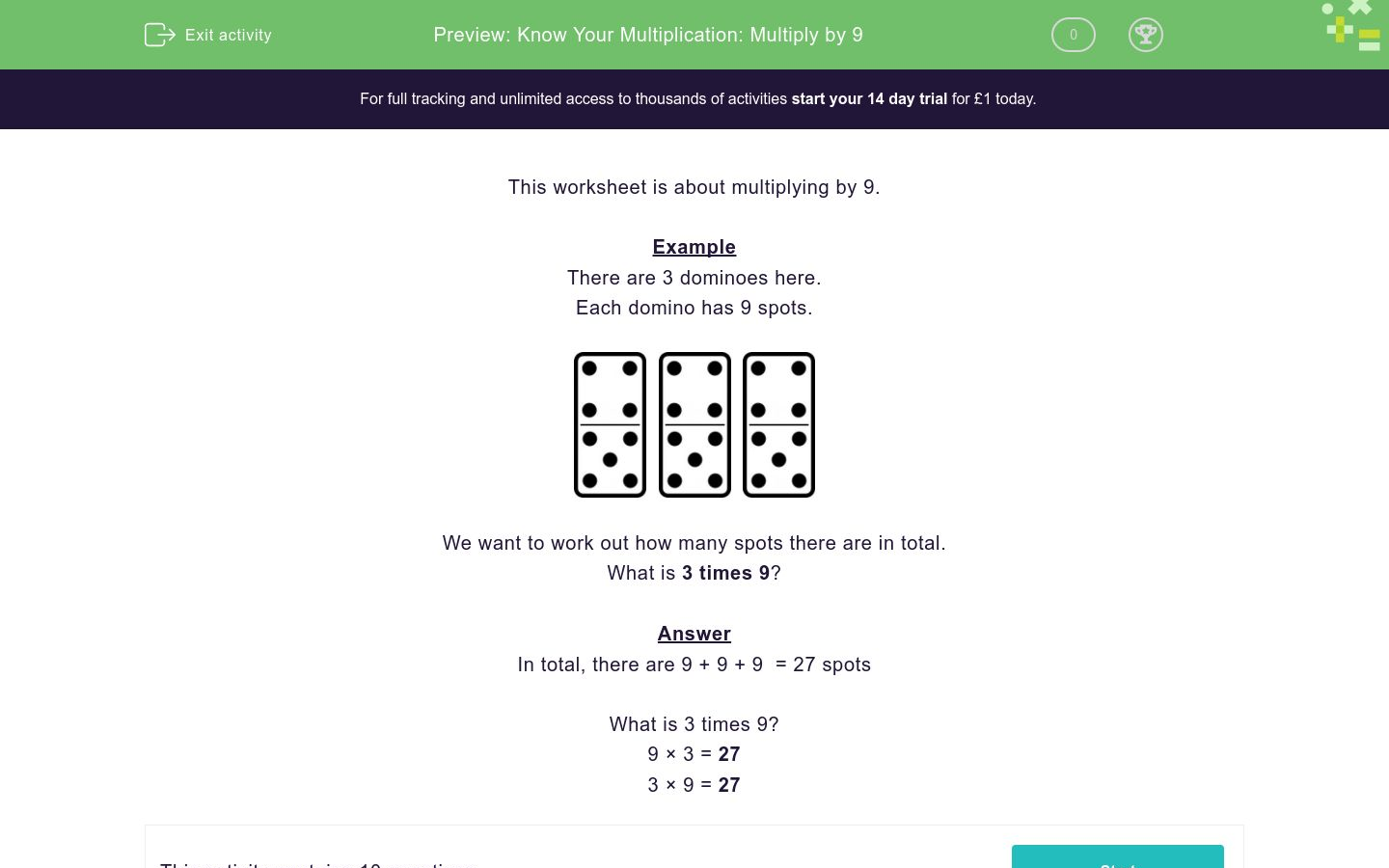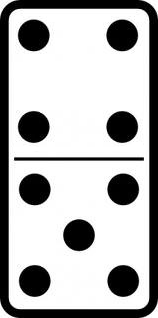# Know Your Multiplication: Multiply by 9

In this worksheet, students multiply by 9 to find answers to questions.Key stage:  KS 2

Curriculum topic:   Number: Multiplication and Division

Curriculum subtopic:   Know Multiplication Tables up to 12 x 12

Difficulty level:### QUESTION 1 of 10

This worksheet is about multiplying by 9.

Example

There are 3 dominoes here.

Each domino has 9 spots.We want to work out how many spots there are in total.

What is 3 times 9?

In total, there are 9 + 9 + 9  = 27 spots

What is 3 times 9?

9 × 3 = 27

3 × 9 = 27

Here are 3 planters with flowers.

Each planter has 9 flowers.We want to work out how many flowers there are in total.

What is 3 times 9?

Here are 7 dominoes.

Each domino has 9 spots.We want to work out how many spots there are in total.

What is 7 times 9?

Here are 6 planters with flowers.

Each planter has 9 flowers.We want to work out how many flowers there are in total.

What is 6 times 9?

What is 2 times 9?

Here are 9 planters with flowers.

Each planter has 9 flowers.We want to work out how many flowers there are in total.

What is 9 times 9?

What is 3 times 9?

Here are 8 dominoes.

Each domino has 9 spots.We want to work out how many spots there are in total.

What is 8 times 9?

Here are 4 planters with flowers.

Each planter has 9 flowers.We want to work out how many flowers there are in total.

What is 4 times 9?

What is 10 times 9?

What is 1 times 9?

• Question 1

Here are 3 planters with flowers.

Each planter has 9 flowers.We want to work out how many flowers there are in total.

What is 3 times 9?

27
EDDIE SAYS

3 x 9 = 27

9 + 9 + 9 = 27

• Question 2

Here are 7 dominoes.

Each domino has 9 spots.We want to work out how many spots there are in total.

What is 7 times 9?

63
EDDIE SAYS

7 x 9 = 63

9 + 9 + 9 + 9 + 9 + 9 + 9 = 63

• Question 3

Here are 6 planters with flowers.

Each planter has 9 flowers.We want to work out how many flowers there are in total.

What is 6 times 9?

54
EDDIE SAYS

6 x 9 = 54

9 + 9 + 9 + 9 + 9 + 9 = 54

• Question 4

What is 2 times 9?

18
EDDIE SAYS

2 x 9 = 18

9 + 9 = 18

• Question 5

Here are 9 planters with flowers.

Each planter has 9 flowers.We want to work out how many flowers there are in total.

What is 9 times 9?

81
EDDIE SAYS

9 x 9 = 81

9 + 9 + 9 + 9 + 9 + 9 + 9 + 9 + 9 = 81

• Question 6

What is 3 times 9?

27
EDDIE SAYS

3 x 9 = 27

9 + 9 + 9 = 27

• Question 7

Here are 8 dominoes.

Each domino has 9 spots.We want to work out how many spots there are in total.

What is 8 times 9?

72
EDDIE SAYS

8 x 9 = 72

9 + 9 + 9 + 9 + 9 + 9 + 9 + 9 = 72

• Question 8

Here are 4 planters with flowers.

Each planter has 9 flowers.We want to work out how many flowers there are in total.

What is 4 times 9?

36
EDDIE SAYS

4 x 9 = 36

9 + 9 + 9 + 9 = 36

• Question 9

What is 10 times 9?

90
EDDIE SAYS

10 x 9 = 90

9 + 9 + 9 + 9 + 9 + 9 + 9 + 9 + 9 + 9 = 90

• Question 10

What is 1 times 9?

9
EDDIE SAYS

1 x 9 = 9

---- OR ----

Sign up for a £1 trial so you can track and measure your child's progress on this activity.

### What is EdPlace?

We're your National Curriculum aligned online education content provider helping each child succeed in English, maths and science from year 1 to GCSE. With an EdPlace account you’ll be able to track and measure progress, helping each child achieve their best. We build confidence and attainment by personalising each child’s learning at a level that suits them.

Get started